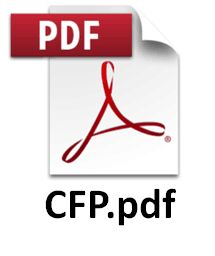Call for PapersTAMC 2020 aims at bringing together a wide range of researchers with interest in computational theory, information theory and applications. The main themes of the conference are computability, complexity, algorithms, information theory and their extensions to machine learning theory, and foundations of artificial intelligence. The topics of interest include (but are not limited to) the following:

• AI modeling
• algebraic computation
• algorithmic coding and number theory
• algorithmic graph theory and combinatorics
• algorithms and data structures
• approximation algorithms
• automata theory
• computational biology and biological computing
• computational complexity
• computational game theory
• computational geometry
• computer science logic
• continuous and real computation
• cryptography
• domain models
• dynamical algorithms
• foundations of artificial intelligence
• game theory
• information theoretical foundation for artificial intelligence
• learning theory, and intelligent computing
• model theory for computing
• network theory and communication networks
• online algorithms
• optimization
• parameterized algorithms
• privacy and security
• process models
• programming and verification
• property testing
• quantum computing
• randomized algorithms
• randomness and pseudo-randomness
• streaming algorithms
• structural information theory
• systems theory
• Turing definability
• VLSI models of computation

The proceedings of the Conference will be published by Springer-Verlag in the Lecture Notes in Computer Science (LNCS) series, and will be available for distribution at the conference. Selected high quality papers will be invited to one of three special issues of journals, Theoretical Computer Science, Mathematical Structures in Computer Science, and SCIENCE CHINA Information Sciences. The invited papers will go through a normal reviewing process.

Important Dates
 Submission deadline: December 1, 2019 (AoE) Notification of acceptance: January 20, 2020 Camera-ready and registration: February 10, 2020 Conference dates: May 23-25, 2020

Submission Instructions
The submission Web page for TAMC 2020 is https://easychair.org/conferences/?conf=tamc2020.

Contact Information
tamc2020@csu.edu.cn